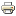Sales and technical support 07572 532 884# What is three phase AC?Printer-friendly version

In a three-phase network there are three live wires called L1, L2, and L3 and a potential difference exists between each of these and neutral.

If a potential difference of 240 Vrms exists between each phase and neutral, and if the phases oscillate at the same frequency, and if the frequency of each phase is offset by a constant and equal amount from each of the other two phases then the waveform looks like a succession of overlapping sinusoids. This can also be drawn in vector form except that now each of the three phases occurs at 0, 120, and 240 degrees around the neutral.

The magic thing is that there is also a potential difference between L1 and L2, and L1 and L3, and L2 and L3, and simple maths reveals that the voltage between phases is 415 Vrms and that this voltage also varies as a sinusoid (the formula is 240 V = 415 V / √3).

UK electrical distribution systems are set up so that three-phase 415 V phase-to-phase is provided to industrial customers whereas domestic customers are provided with single-phase (N-L) 240 V from the same network. This is the system in the UK - see below for other countries.